# C - fscanf

## File Operations in TamilLearn C - C tutorial - C fscanf - C examples - C programs

## C - fscanf() - Definition and Usage

• In C – Programming, the fscanf function is used to read formatted input from a stream.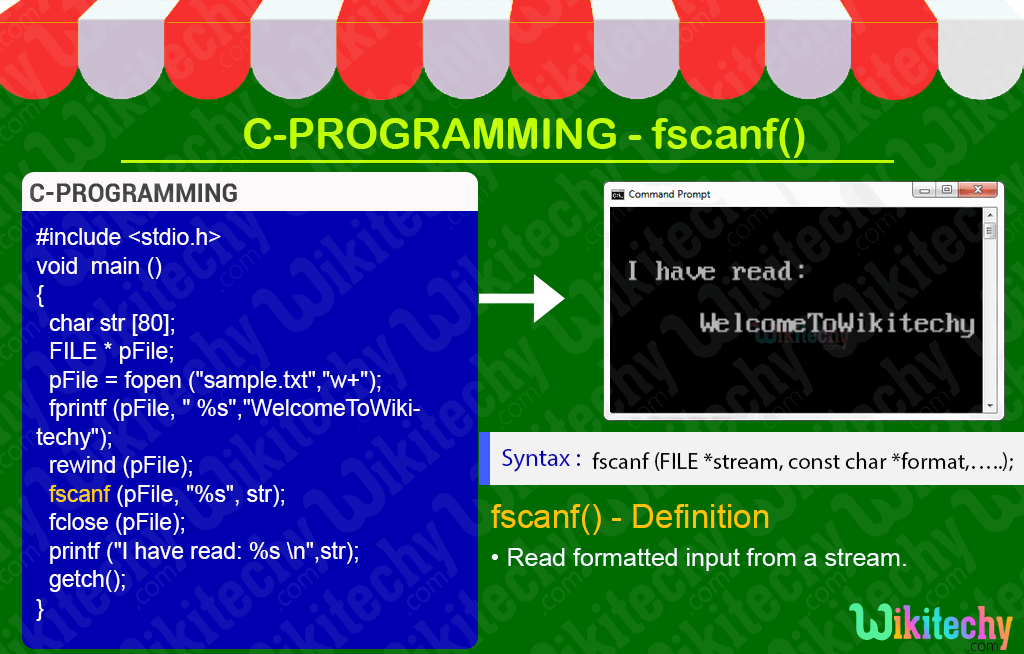## C Syntax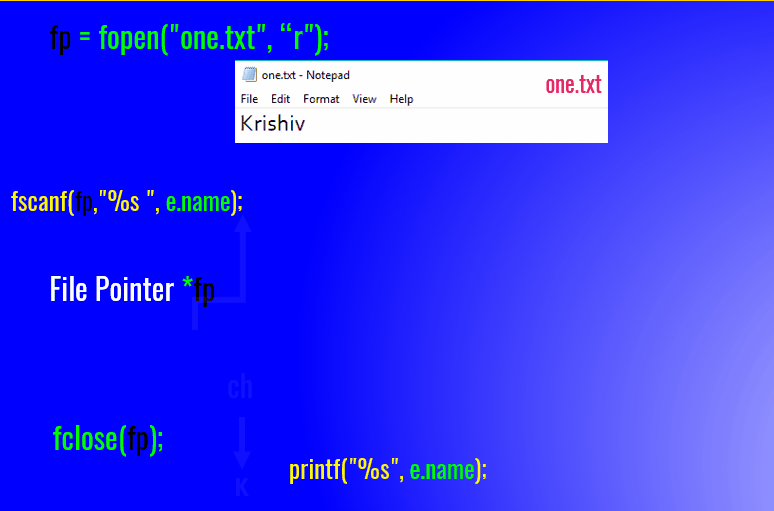## C Code - Explanation :

1. In this statement we create the character array and the variable name as “str”.
2. Here in this statement we create a file pointer “pFile”. Here in this statement we open the text file using “fopen” function in writing mode.
3. In this statement we write the content in the text file using fprintf function.
4.5. In this statement we use the rewind function used to find the position of the string.
6. In this statement we read the input string using fscanf function.
7. After the file manipulation the file will be closed using fclose function.
8. Here in this printf statement we print the text file as string.

## Sample Output - Programming Examples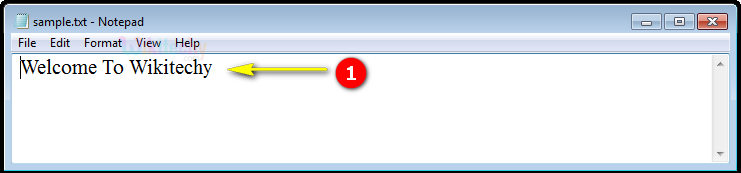1. Here in this output the written statement “WelcomeToWikitechy” in the sample.txt file using fprintf function as shown here.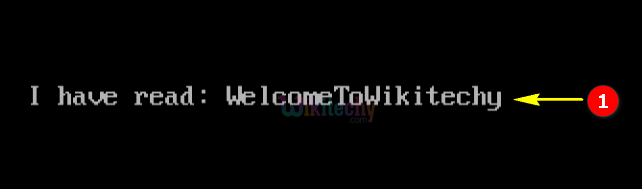1. Here in this output, the statement (“WelcomeToWikitechy”) which has been read in the sample.txt file using fscanf function as shown here.

## Output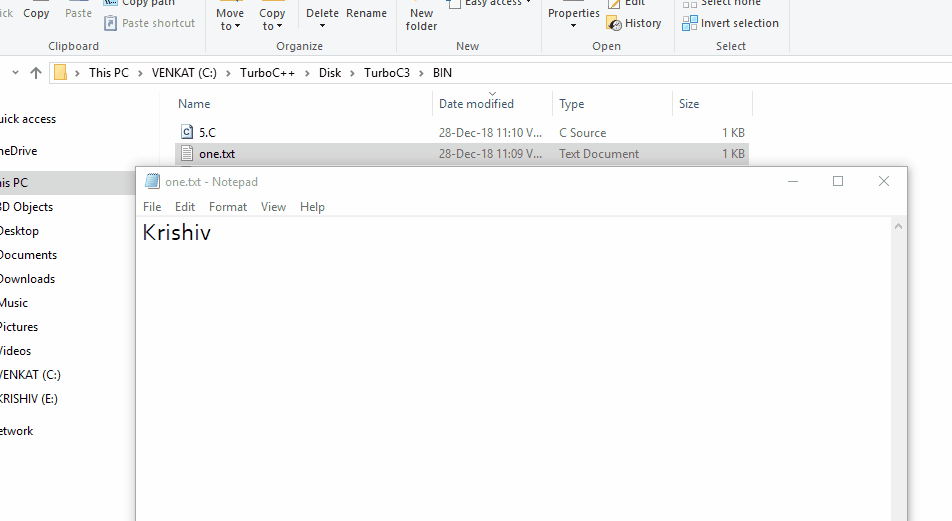Learn C - C tutorial - C fscanf - C examples - C programs